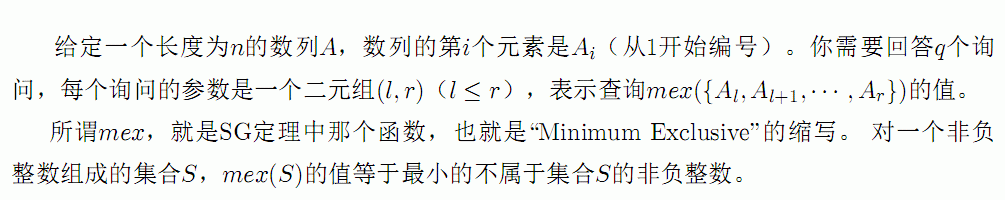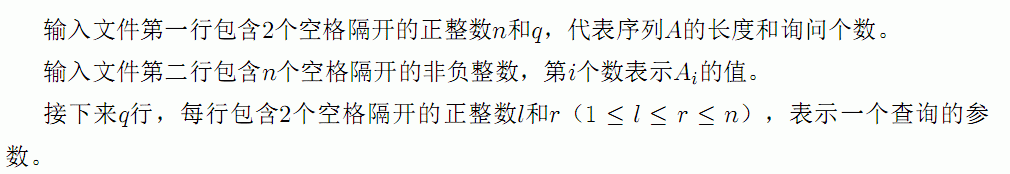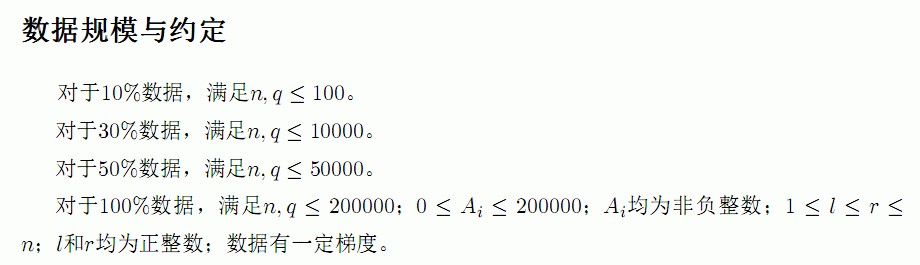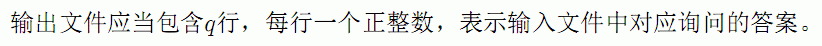DescriptionInputOutputSolutions

这么经典的题居然拖到现在才写QAQ。

假设我们已经知道了区间$[x,y]$的答案。现在考虑左指针右移产生的影响，即$[x,y]$区间的答案对$[x+1,y]$的影响。

左指针右移，最直接的影响就是$a_x$元素减少。如果$[x+1,y]$区间内依旧有$a_x$元素，那答案显然不会更新。反之，如果区间内不存在$a_x$元素，那这个区间答案可能被$a_x$更新，此时答案为$min(ans,a_x)$。

这个思想可以扩展到正解上。预处理出$[1,x],x \leq n$的所有答案，这个$O(N)$可解决。然后考虑指针右移，答案有可能更新的区间显然为$[i,next(a_i))$，这是一个区间取$min$操作，线段树可以实现。

然后将所有操作离线处理，保证左指针单调后，就可以处理所有答案了。

这题还可以利用主席树实现在线询问。

我们想要对每个前缀构造出一个权值线段树，使得答案能够通过在这棵树上二分查找求解。这个显然是主席树的作用。

具体做法是，对每个前缀维护权值区间内的最小出现位置。然后对于每个询问$[x,y]$，其实就是在$[1,y]$前缀的权值线段树内寻找第一个出现位置$<x$的数字，二分下去即可。